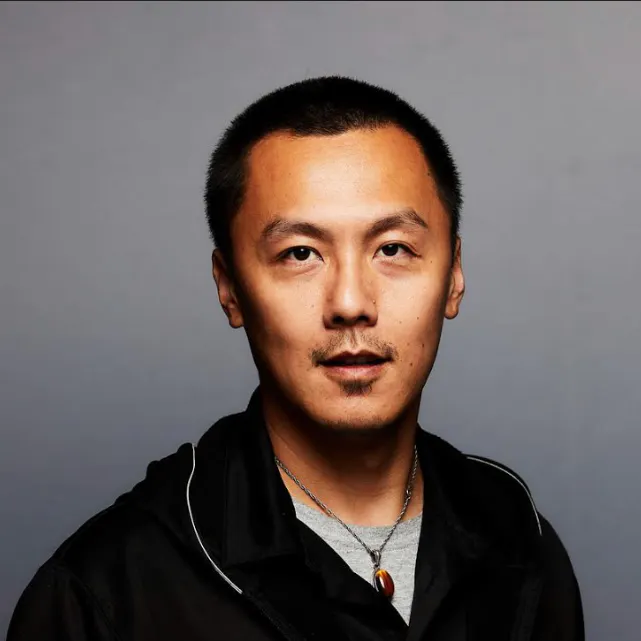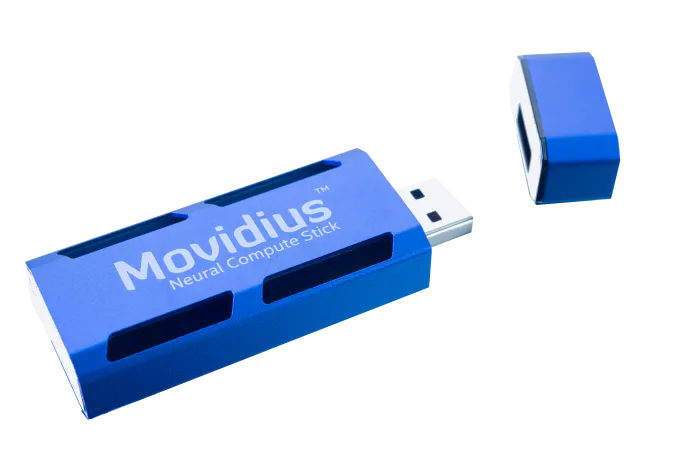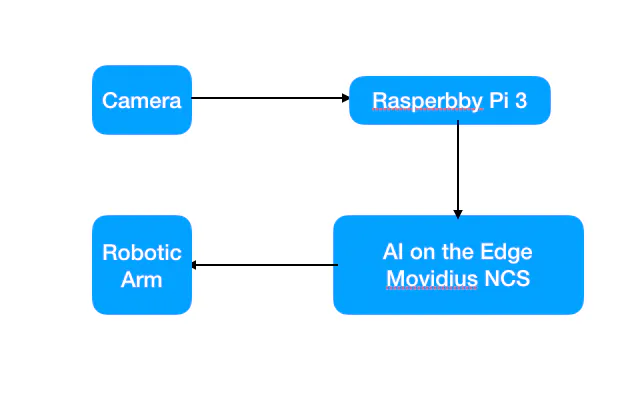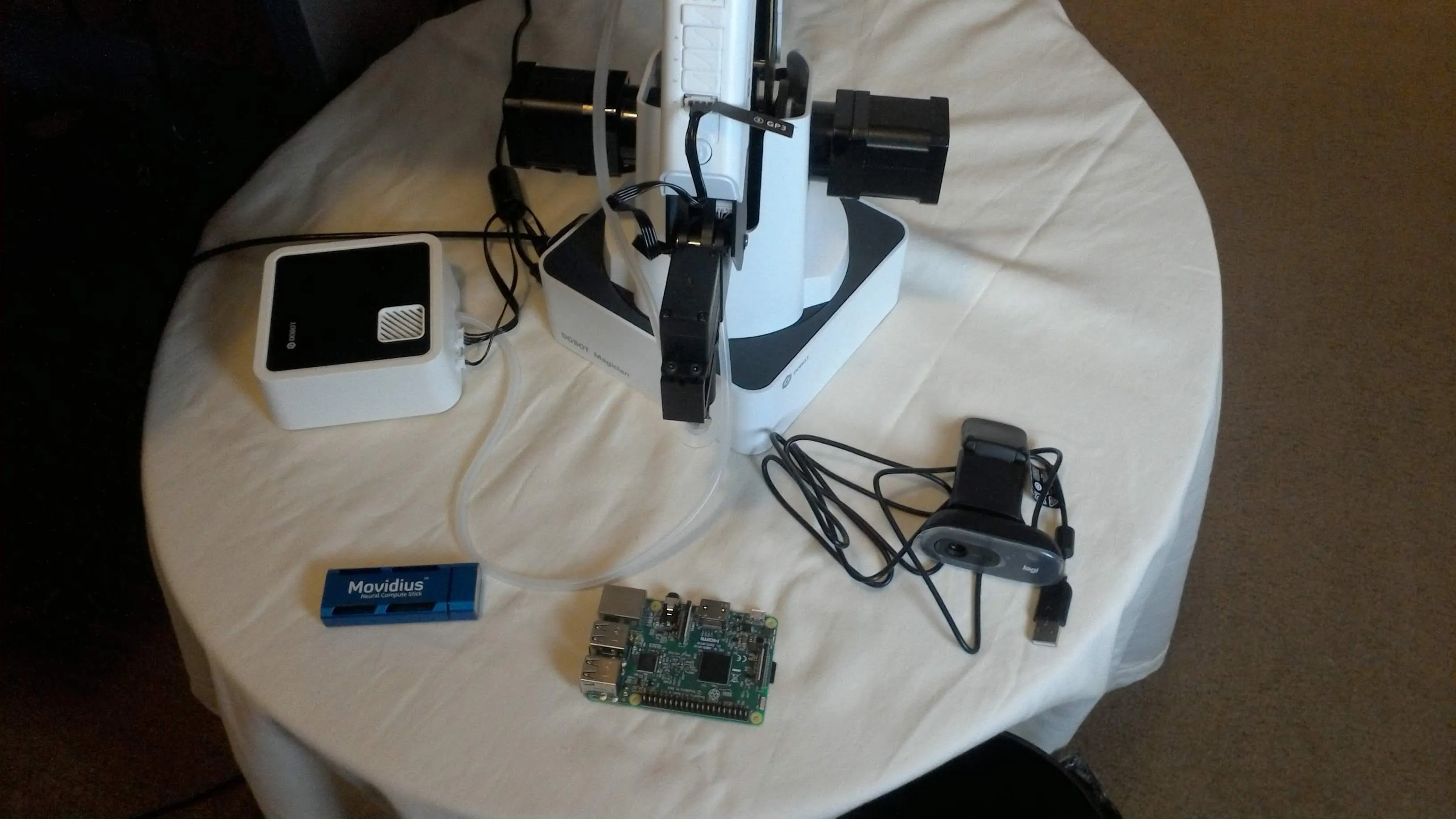# Trash and Recyclable Sorting Robot Arm

Using AI on the edge to classify recycle vs. landfill and use robotic arm for sorting the garbage.

## Things used in this project

### Hardware components

 Dobot Magician
×1Raspberry Pi 3 Model B
×1Intel Movidius Neural Compute Stick
×1

Raspberry Pi

## Schematics

### Overall Architecture

Overall Architecture### Materials needed

Materials needed for this## Code

### Robot Arm movement file

Python
Arm file used to move robotic arm
```import threading
import DobotDllType as dType
import time
import argparse
import json
from pprint import pprint
from collections import OrderedDict
class ScriptRobot():
global CON_STR
CON_STR = {
dType.DobotConnect.DobotConnect_NoError:  "DobotConnect_NoError",
dType.DobotConnect.DobotConnect_NotFound: "DobotConnect_NotFound",
dType.DobotConnect.DobotConnect_Occupied: "DobotConnect_Occupied"}
def __init__(self,Json):
self.Json=Json
self.state=""
def Connect(self):
#Connect Dobot
self.state = dType.ConnectDobot(self.api, "", 115200)
dType.GetDeviceSN(self.api)
dType.GetDeviceName(self.api)
dType.GetDeviceVersion(self.api)
dType.GetDeviceWithL(self.api)
dType.GetPoseL(self.api)
dType.GetKinematics(self.api)
#dType.GetHOMEParams(self.api)
print("Connect status:",CON_STR[self.state])
if (self.state == dType.DobotConnect.DobotConnect_NoError):
dType.SetQueuedCmdClear(self.api)
return True
else :
dType.DisconnectDobot(self.api)
return False
"""
def _MOVJ(self,data):
dType.SetPTPCmd(self.api,dType.PTPMode.PTPMOVJXYZMode,float(data['X']),float(data['Y']),float(data['Z']),float(data['R']), isQueued = 1)
def _MOVL(self,data):
dType.SetPTPCmd(self.api,dType.PTPMode.PTPMOVLXYZMode,float(data['X']),float(data['Y']),float(data['Z']),float(data['R']), isQueued = 1)
def _ARC(self,value,data):
dType.SetARCCmd(self.api,[float(data['X']),float(data['Y']),float(data['Z']),0],[float(data['_X']),float(data['_Y']),float(data['_Z']),0], isQueued = 1)
def moveTypes(self,value,data):
if value=="MOVJ" :
return self._MOVJ(data)
elif value=="MOVL" :
return self._MOVL(data)
elif value=="ARC" :
return self._ARC(value,data)
"""
def ParserMove(self):
dType.SetQueuedCmdClear(self.api)
json_data = open(self.Json)
#def SetPTPCoordinateParams(api, xyzVelocity, xyzAcceleration, rVelocity,  rAcceleration,  isQueued=0):
for move in data:
#print "TEST_:"+data[move]['MotionStyle'],data[move]['Row']
if data[move]['PauseTime']!=0:
lastIndex=dType.SetWAITCmd(self.api,float(data[move]['PauseTime']), isQueued = 1)
if data[move]['MotionStyle']=="MOVJ" :
lastIndex=dType.SetPTPCmd(self.api,dType.PTPMode.PTPMOVJXYZMode,float(data[move]['X']),float(data[move]['Y']),float(data[move]['Z']),float(data[move]['R']), isQueued = 1)
dType.SetEndEffectorSuctionCup(self.api, 1, 0, isQueued = 1)
if data[move]['MotionStyle']=="MOVL" :
lastIndex=dType.SetPTPCmd(self.api,dType.PTPMode.PTPMOVLXYZMode,float(data[move]['X']),float(data[move]['Y']),float(data[move]['Z']),float(data[move]['R']), isQueued = 1)
if data[move]['MotionStyle']=="MOVJANGLE" :
lastIndex=dType.SetARCCmd(self.api,[float(data[move]['X']),float(data[move]['Y']),float(data[move]['Z']),0],[float(data[move]['_X']),float(data[move]['_Y']),float(data[move]['_Z']),0], isQueued = 1)
dType.SetQueuedCmdStartExec(self.api)
while lastIndex > dType.GetQueuedCmdCurrentIndex(self.api):
#           dType.GetPose(self.api) Obtener la Posicion actual del robot
dType.dSleep(100)
dType.SetQueuedCmdStopExec(self.api)
dType.GetKinematics(self.api)
dType.DisconnectDobot(self.api)
if __name__ == "__main__":
parser = argparse.ArgumentParser(description='Script Robot')
parser.add_argument('--Json', required=True, help='File name export json')
args = parser.parse_args()
R = ScriptRobot(args.Json)
R.Connect()
R.ParserMove()
```

### AI On the Edge

Python
Movidius Neural Computing stick for running live object detection
```#!/usr/bin/python3

# ****************************************************************************
# ****************************************************************************

# Detect objects on a LIVE camera feed using
# Intel® Movidius™ Neural Compute Stick (NCS)

import os
import cv2
import sys
import numpy
import ntpath
import argparse
import subprocess

import mvnc.mvncapi as mvnc

from utils import visualize_output
from utils import deserialize_output

# Detection threshold: Minimum confidance to tag as valid detection
CONFIDANCE_THRESHOLD = 0.60 # 60% confidant

# Variable to store commandline arguments
ARGS                 = None

# OpenCV object for video capture
camera               = None

framecount = 0
# ---- Step 1: Open the enumerated device and get a handle to it -------------

def open_ncs_device():

# Look for enumerated NCS device(s); quit program if none found.
devices = mvnc.EnumerateDevices()
if len( devices ) == 0:
print( "No devices found" )
quit()

# Get a handle to the first enumerated device and open it
device = mvnc.Device( devices )
device.OpenDevice()

return device

# ---- Step 2: Load a graph file onto the NCS device -------------------------

# Read the graph file into a buffer
with open( ARGS.graph, mode='rb' ) as f:

# Load the graph buffer into the NCS
graph = device.AllocateGraph( blob )

return graph

# ---- Step 3: Pre-process the images ----------------------------------------

def pre_process_image( frame ):

# Resize image [Image size is defined by choosen network, during training]
img = cv2.resize( frame, tuple( ARGS.dim ) )

# Convert RGB to BGR [OpenCV reads image in BGR, some networks may need RGB]
if( ARGS.colormode == "rgb" ):
img = img[:, :, ::-1]

# Mean subtraction & scaling [A common technique used to center the data]
img = img.astype( numpy.float16 )
img = ( img - numpy.float16( ARGS.mean ) ) * ARGS.scale

return img

# ---- Step 4: Read & print inference results from the NCS -------------------

def infer_image( graph, img, frame ):

# Load the image as a half-precision floating point array

# Get the results from NCS
output, userobj = graph.GetResult()

# Get execution time
inference_time = graph.GetGraphOption( mvnc.GraphOption.TIME_TAKEN )

# Deserialize the output into a python dictionary
output_dict = deserialize_output.ssd(
output,
CONFIDANCE_THRESHOLD,
frame.shape )

# Print the results (each image/frame may have multiple objects)
#print( "I found these objects in "
#        + " ( %.2f ms ):" % ( numpy.sum( inference_time ) ) )

global framecount
for i in range( 0, output_dict['num_detections'] ):
#print( "%3.1f%%\t" % output_dict['detection_scores_' + str(i)]
#      + labels[ int(output_dict['detection_classes_' + str(i)]) ]
#     + ": Top Left: " + str( output_dict['detection_boxes_' + str(i)] )
#     + " Bottom Right: " + str( output_dict['detection_boxes_' + str(i)] ) )

if 'bottle' in labels[ int(output_dict['detection_classes_' + str(i)]) ] and framecount < 8:
framecount = framecount + 1
# Draw bounding boxes around valid detections
(y1, x1) = output_dict.get('detection_boxes_' + str(i))
(y2, x2) = output_dict.get('detection_boxes_' + str(i))

# Prep string to overlay on the image
display_str = (
labels[output_dict.get('detection_classes_' + str(i))]
+ ": "
+ str( output_dict.get('detection_scores_' + str(i) ) )
+ "%" )

frame = visualize_output.draw_bounding_box(
y1, x1, y2, x2,
frame,
thickness=4,
color=(255, 255, 0),
display_str=display_str )
elif 'bottle' in labels[ int(output_dict['detection_classes_' + str(i)]) ] and framecount > 5:
framecount = 0
os.system('python3 Script.py --Json garbage.json')
print('called robot')

if 'tvmonitor' in labels[ int(output_dict['detection_classes_' + str(i)]) ] and framecount < 8:
framecount = framecount + 1
# Draw bounding boxes around valid detections
(y1, x1) = output_dict.get('detection_boxes_' + str(i))
(y2, x2) = output_dict.get('detection_boxes_' + str(i))

# Prep string to overlay on the image
display_str = (
labels[output_dict.get('detection_classes_' + str(i))]
+ ": "
+ str( output_dict.get('detection_scores_' + str(i) ) )
+ "%" )

frame = visualize_output.draw_bounding_box(
y1, x1, y2, x2,
frame,
thickness=4,
color=(255, 255, 0),
display_str=display_str )
elif 'tvmonitor' in labels[ int(output_dict['detection_classes_' + str(i)]) ] and framecount > 5:
framecount = 0
os.system('python3 Script.py --Json recycle.json')
print('called robot')

print( '\n' )

# If a display is available, show the image on which inference was performed
if 'DISPLAY' in os.environ:
cv2.imshow( 'NCS live inference', frame )

# ---- Step 5: Unload the graph and close the device -------------------------

def close_ncs_device( device, graph ):
graph.DeallocateGraph()
device.CloseDevice()
camera.release()
cv2.destroyAllWindows()

# ---- Main function (entry point for this script ) --------------------------

def main():

device = open_ncs_device()

# Main loop: Capture live stream & send frames to NCS
while( True ):
img = pre_process_image( frame )
infer_image( graph, img, frame )

# Display the frame for 5ms, and close the window so that the next
# frame can be displayed. Close the window if 'q' or 'Q' is pressed.
if( cv2.waitKey( 5 ) & 0xFF == ord( 'q' ) ):
break

close_ncs_device( device, graph )

# ---- Define 'main' function as the entry point for this script -------------

if __name__ == '__main__':

parser = argparse.ArgumentParser(
description="Detect objects on a LIVE camera feed using \
Intel® Movidius™ Neural Compute Stick." )

default='../../caffe/SSD_MobileNet/graph',
help="Absolute path to the neural network graph file." )

default=0,
help="Index of your computer's V4L2 video device. \
ex. 0 for /dev/video0" )

default='../../caffe/SSD_MobileNet/labels.txt',
help="Absolute path to labels file." )

nargs='+',
default=[127.5, 127.5, 127.5],
help="',' delimited floating point values for image mean." )

default=0.00789,
help="Absolute path to labels file." )

nargs='+',
default=[300, 300],
help="Image dimensions. ex. -D 224 224" )

default="bgr",
help="RGB vs BGR color sequence. This is network dependent." )

ARGS = parser.parse_args()

# Create a VideoCapture object
camera = cv2.VideoCapture( ARGS.video )

# Set camera resolution
camera.set( cv2.CAP_PROP_FRAME_WIDTH, 620 )
camera.set( cv2.CAP_PROP_FRAME_HEIGHT, 480 )

labels =[ line.rstrip('\n') for line in
open( ARGS.labels ) if line != 'classes\n']

main()

# ==== End of file ===========================================================
```

library for Pi

## Credits

### Peter Ma

44 projects • 293 followers
Prototype Hacker, Hackathon Goer, World Traveler, Ecological balancer, integrationist, technologist, futurist.